# ODE Runge Kutta 4th Order VI

## LabVIEW 2018 Help

Edition Date: March 2018
Part Number: 371361R-01
View Product InfoLabVIEW 2016 HelpLabVIEW 2017 HelpLabVIEW 2018 HelpLabVIEW 2019 HelpLabVIEW 2020 Help

Owning Palette: Ordinary Differential Equations VIs

Requires: Full Development System

Solves ordinary differential equations with initial conditions using the Runge Kutta method.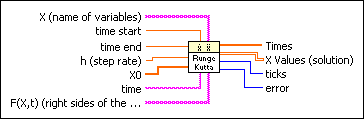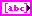X is an array of strings of variables.time start is the start point of the ODE. The default is 0.time end is the end point of the time interval under investigation. The default is 1.0.h is the fixed step rate. The default is 0.1.X0 is the vector of the start condition x, …, x[n0]. There is a one-to-one relation between the components of X0 and X.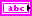time is the string denoting the time variable. The default variable is t.F(X,t) is a 1D array of strings representing the right sides of the differential equations. The formulas can contain any number of valid variables.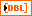Times is an array representing the time steps. The Runge Kutta method yields equidistant time steps between time start and time end.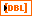X Values is a 2D array of the solution vector x, …, x[n]. The top index runs over the time steps, as specified in the Times array, and the bottom index runs over the elements of x, …, x[n].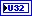ticks is the time in milliseconds for the whole calculation.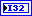error returns any error or warning from the VI. Errors are produced by using the wrong inputs X, X0, and F(X,t). You can wire error to the Error Cluster From Error Code VI to convert the error code or warning into an error cluster.

## ODE Runge Kutta 4th Order Details

The Runge Kutta method of 4th order works with a higher degree of accuracy than the common Euler method and with a fixed step rate as a five stage process, more precisely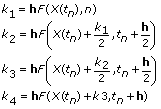and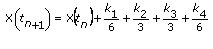with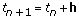The method ends if

tntime end.

The following illustration shows the solution of the following system of ordinary differential equations: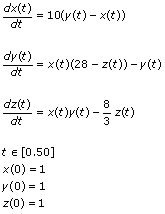Enter the following equations on the front panel:

• time start: 0.00
• time end: 50.00
• X: [x, y, z]
• X0: [1, 1, 1]
• F(X,t): [10*(y - x), x*(28 - z) - y, x*y - (8/3)*z]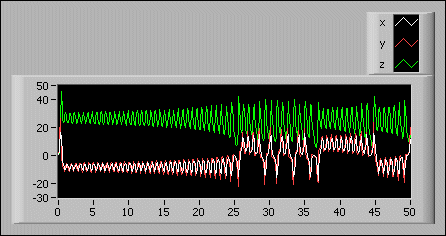Note  Even though there are actually three solutions, a first glance at the graph almost seems to show only two solutions. This is because the solutions for x and y are very similar, so they almost overlap.

## Examples

Refer to the following VIs for examples of using the ODE Runge Kutta 4th Order VI:

• Shooting Method VI: labview\examples\Mathematics\Differential Equations - ODE
• Process Control Explorer VI: labview\examples\Mathematics\Differential Equations - ODE